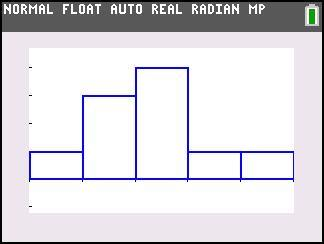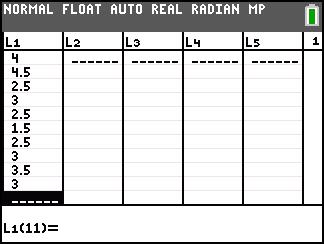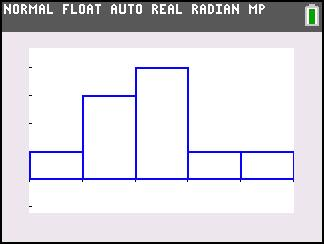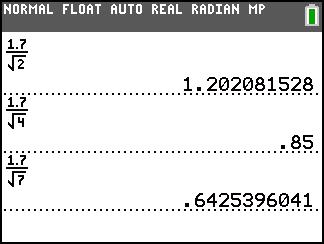••• ##### Device
• TI-83 Plus Family
• TI-84 Plus
• TI-84 Plus Silver Edition
•TI-84 Plus C Silver Edition
•TI-84 Plus CE

# Statistics: Intro to the Central Limit Theorem

by Texas Instruments#### Overview

Students discover the Central Limit Theorem by simulating rolls of two, four, and seven number cubes via the random number generator.

#### Key Steps

•In problem 1, students are grouped by threes. The first person simulates rolling two number cubes 10 times, saying the results of each roll aloud. The second person will calculate the means of the two numbers on the Home screen. The third person will record the means, both in the List Editor.

•Students will then perform a simulation of rolling four and seven number cubes, finding the means, and recording them in the spreadsheet and on the worksheet. After each simulation, students will create a histogram.

•The groups finish, they discuss the answers to the questions. They will see that in the Central Limit Theorem (CLT) the distribution of sample means becomes normal as n increases, regardless of the shape of the distribution of the population. It could also be normal, skewed, bimodal, or have no pattern. At the end of this activity, students will be able to state the Central Limit Theorem.